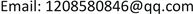1. 引言

2. 小波包降噪

1) 选择一个小波基并确定分解层次N，然后对信号进行N层小波包分解；

2) 计算最佳树(即确定最佳小波包基)，对一个给定的熵标准，计算最佳小波包分解树；

3) 对各个分解尺度下的高频系数选择一个适当的阈值进行阈值量化处理。为得到最优结果以满足特定的分析和信息评价标准，阈值通过反复实验不断调整来确定；

4) 根据第N层的小波包分解低频系数和量化处理系数进行小波重构。

3. 变分模式分解(VMD)

VMD是一种通过搜寻约束变分模型最优解来分解信号并得到本征模态分量(IMF)的自适应分解方法。其实质是变分问题的构造和求解   。

3.1. 构造变分问题

1) 用希尔伯特方法对各个IMF进行处理得到解析信号；

( δ ( t ) + j π t ) ∗ y k ( t ) (1)

2) 混合一个预估的中心频率 w k 并移频。

[ ( δ ( t ) + j π t ) ∗ y k ( t ) ] e − j w k t (2)

3) 计算梯度的平方范数即对解调信号做高斯平滑处理，则约束变分问题可表示为：

{ min { y k } , { w k } { ∑ k = 1 K ‖ ∂ t [ ( δ ( t ) + j π t ) ∗ y k ( t ) ] e − j w k t ‖ 2 2 } S .t .   ∑ k = 1 K y k ( t ) = y ( t ) (3)

3.2. 求解变分问题

ς ( { y k } , { w k } , λ ) = α ∑ k ‖ ∂ t [ ( δ ( t ) + j π t ) y k ( t ) ] e − j w k t ‖ 2 2     + ‖ y ( t ) − ∑ k y k ( t ) ‖ 2 2 + 〈 λ ( t ) , y ( t ) − ∑ k y k ( t ) 〉 (4)

VMD运用了乘法算子交替方向法，通过轮替更新 y k n + 1 ， w k n + 1 和 λ n + 1 求得拉格朗日表达式的“鞍点”。变分问题的解 y k n + 1 ( t ) 在频率内表示如下：

Y ^ k n + 1 ( w ) = Y ^ ( w ) − ∑ i ≠ k Y ^ i ( w ) + λ ^ ( w ) 2 1 + 2 α ( w − w k ) 2 (5)

w k n + 1 ( w ) = ∫ 0 ∞ w | Y ^ k ( w ) | 2 d w ∫ 0 ∞ | Y ^ k ( w ) | 2 d w (6)

4. 实验分析

1) 首先，使用小波包方法对信号进行降噪处理，本文选用小波包基函数为db7，分解层数为3层，对分解后所得的8个小波包节点采用默认阈值降噪，后将处理后各个节点进行重构后形成降噪后信号。图4为滚动轴承降噪后信号。从图易见，图4中信号谱线比图3中谱线更为清晰，且通过计算得到降噪后信号信噪比达到4.5124，说明小波包方法有效滤除了滚动轴承振动信号中的噪声成分，凸显出了信号的有用成分。

2) 为将降噪后信号中各组成成分进行划分，对降噪后信号做VMD分解，其中VMD方法中根据中心频率相近原则设定模态数K = 5，惩罚因子 α = 1500 ，拉格朗日乘子 λ = 0 ，图5为VMD分解后所得部分IMF分量。从图可以看出，信号的各成分被清晰的分解到了各个不同的频带上，有效的将故障成分与设备固有振动等成分进行了区分。

3) 将分解后所得各IMF分量与降噪后信号做互相关分析，选取相关系数最大即包含有信号最多有用信息(即最多故障冲击成分)的IMF2分量进行Hilbert包络解调变换，得到图6所示结果。从图中可以看出，谱线中105.5 Hz处出现明显的波峰值，与滚动轴承外圈故障特征频率基本吻合。且在故障频率的2倍频210.9 Hz及3倍频316.4 Hz处也出现了较为清晰的波峰，Hilbert包络解调方法成功提取出了故障特征频率。

5. 结论